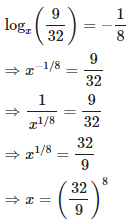Courses

# Test: Logarithm- 2

## 10 Questions MCQ Test Quantitative Aptitude (Quant) | Test: Logarithm- 2

Description
This mock test of Test: Logarithm- 2 for Quant helps you for every Quant entrance exam. This contains 10 Multiple Choice Questions for Quant Test: Logarithm- 2 (mcq) to study with solutions a complete question bank. The solved questions answers in this Test: Logarithm- 2 quiz give you a good mix of easy questions and tough questions. Quant students definitely take this Test: Logarithm- 2 exercise for a better result in the exam. You can find other Test: Logarithm- 2 extra questions, long questions & short questions for Quant on EduRev as well by searching above.
QUESTION: 1

### Which of the following statements is not correct?

Solution: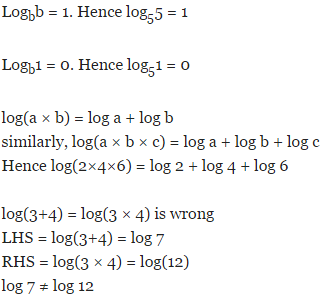QUESTION: 2

Solution:
QUESTION: 3

###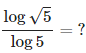Solution: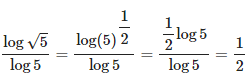QUESTION: 4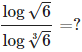Solution: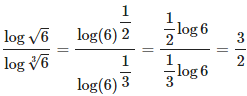QUESTION: 5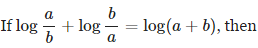Solution: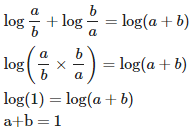QUESTION: 6

If log(64)= 1.806, log(16) = ?

Solution: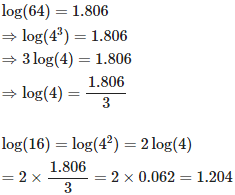QUESTION: 7

If log 2 = 0.3010 and log 3 = 0.4771, What is the value of log51024?

Solution: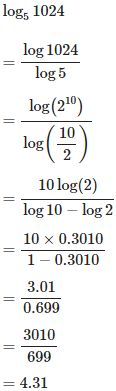QUESTION: 8

if log 2 = 0.30103 and log 3 = 0.4771, find the number of digits in (648)5.

Solution: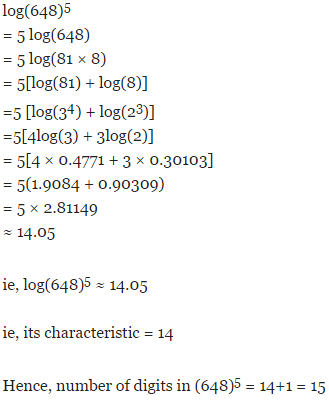QUESTION: 9

if log 2 = 0.30103, the number of digits in 2128 is

Solution:

log(2128) = 128log(2) = 128 × 0.30103 ≈ 38.4

ie, its characteristic = 38

Hence, number of digits in 2128 = 38+1 = 39

QUESTION: 10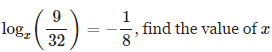Solution: